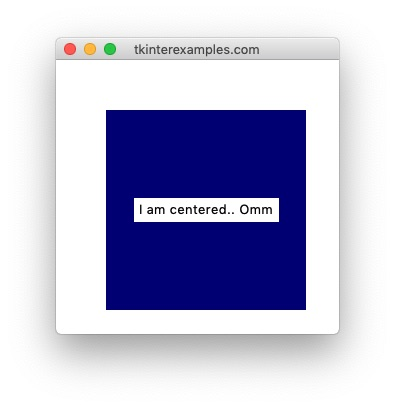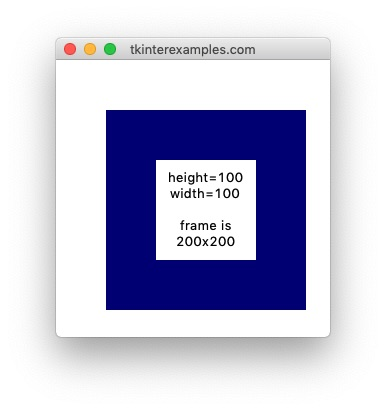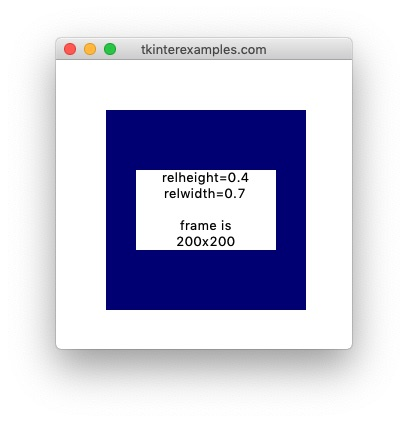# Tkinter Examples

## Place

The `place` geometry manager offers the most precision in element placement out of the three package managers however it has a much smaller set of use cases as it can create a lot of headaches to get things into the proper shape. For static window applications (no resizing) it can be a good option. We would generally advise against using it unless you're an advanced user that knows they need it for their purposes (creating a custom geometry manager for instance).

### Position

To define the position of elements using the `place` geometry manager we have two options: absolute position aand relative position. We can also combine these for some utility. These measurements are always relative to the widget's parent.

#### Absolute Positio

To use absolute positioning we provide the `x=` and `y=` attributes to `.place()` .

```import tkinter

root = tkinter.Tk()

tkinter.Label(root, text="x=50, y=50").place(x=50, y=50)
tkinter.Label(root, text="x=50, y=100").place(x=50, y=100)
tkinter.Label(root, text="x=150, y=75").place(x=150, y=75)

root.mainloop()
```#### Relative Position

To use relative positioning we provide the `relx=` and `rely=` attributes to `.place()` .

```import tkinter

root = tkinter.Tk()

frame = tkinter.Frame(root, height=200, width=200, bg="navy")
frame.place(x=50, y=50)

tkinter.Label(frame, text="relx=0, rely=0").place(relx=0, rely=0)
tkinter.Label(frame, text="relx=0.4, rely=0.6").place(relx=0.4, rely=0.6)
tkinter.Label(frame, text="relx=1, rely=1").place(relx=1, rely=1)

root.mainloop()
```Notice that in the above example we cannot see the last label. This is because by default the `anchor` attribute is set to NW. This means the element's top left corner is touching the bottom right corner of our frame.

#### Centering

Centering elements with `.place()` is rather simple:

```import tkinter

root = tkinter.Tk()

frame = tkinter.Frame(root, height=200, width=200, bg="navy")
frame.place(x=50, y=50)

tkinter.Label(frame, text="I am centered.. Omm").place(relx=0.5, rely=0.5, anchor=tkinter.CENTER)

root.mainloop()
```### Size

Similar to Position, we have two ways of defining the size of an element, absolutely and relative to its parent.

#### Absolute Size

Size can be set absolutely using the `height` and `width` options on the package manager.

```import tkinter

root = tkinter.Tk()

frame = tkinter.Frame(root, height=200, width=200, bg="navy")
frame.place(x=50, y=50)
label = tkinter.Label(frame, text="height=100\nwidth=100\n\nframe is\n200x200")
label.place(height=100, width=100, relx=0.5, rely=0.5, anchor=tkinter.CENTER)

root.mainloop()

```#### Relative Size

Size can be set relatively using the `relheight` and `relwidth` options on the package manager.

```import tkinter

root = tkinter.Tk()